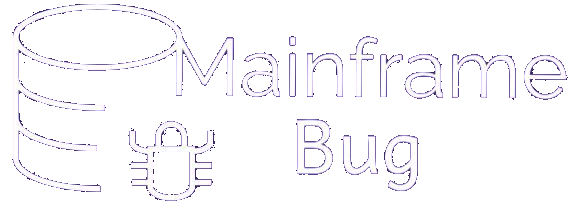## Module 5: Data Division

### Floating point binary number

• It is not a case that numeric data are always integers, they can have fractional part as well. Such a number is actually represented within the computer in two parts, the mantissa and exponent. In computer systems, if n bits are used to represent a number, a bit is used to represent sign, some bit is used to represent the mantissa and the remaining used to represent the exponent. The value is calculated using:-

mantissa * 2exponent

• A number represented in this form is known as floating point binary number.
• In a single precision floating point number, 1 full word(32 bits) is used, out of which first left most bit is used to represent sign, next 7 bits to represent exponent and last (right most) 23 bits are used to represent Mantissa. COBOL COMP-1 phrase uses this representation to store data
• In a double precision floating point number, 1 double word(64 bits) is used, out of which first left most bit is used to represent sign, next 11 bits to represent exponent and last (right most) 52 bits are used to represent Mantissa. COBOL COMP-2 phrase uses this representation to store data